Example Questions

2 Next →

Example Question #2 : How To Use Scientific Notation

What is the result when,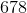,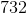is rounded to the nearest thousand and then put in scientific notation?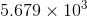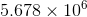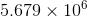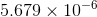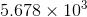Explanation:

First, when we round to the nearest thousand we get 5, 679, 000 since we round up when the next digit is greater than 5.

Then, to put it in scientific notation, we arrange the digits so that a decimal point creates a number between 1 and 10. We get 5.679.

Then, we want the exponent of the 10 to be the number of times the decimal needs to move to the right. This is 6 times.

Thus, we get our answer.Example Question #3 : How To Use Scientific Notation

What is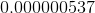in scientific notation?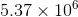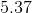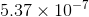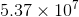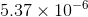Explanation:

In order to write a number in scientific notation, you must shift the number of decimal places to get a number in the ones place.

Since the original number is a decimal, the exponent will need to be negative. This eliminates three answer choices.

In order to getinto scientific notation with '5' in the ones place, you must shift the decimal over seven places.

Therefore, the final answer in scientific notation is.

Example Question #11 : How To Use Scientific Notation

Which of the following is equal to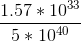?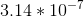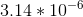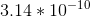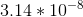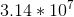Explanation:

For this, you can handle thes separately from the coefficients.  Thes easily cancel: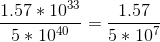Now, handle the coefficients like they are a separate fraction: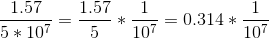Notice, though, that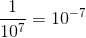: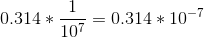Now, you need to get this into scientific notation for your answer.  It is easiest ot think of it like this: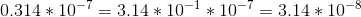Example Question #12 : How To Use Scientific Notation

Simplify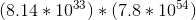.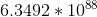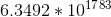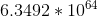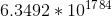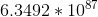Explanation:

The easiest way to work with a large number like this is to combine thefactors and then the other coefficients. After that, you will have to convert the number into scientific notation to match the answer: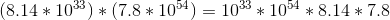Combine the powers of: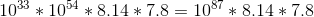Next, combine the other coefficients: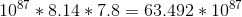Now, rewrite the leading number like this: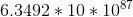Thus, you get:Example Question #13 : How To Use Scientific Notation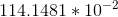is equal to which of the following?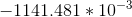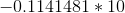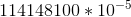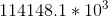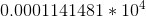Explanation:

The easiest way to solve a question like this is to convert the answers all into easily comparable numbers. Remember that you move the decimal place to the left for negative powers ofand to the right for positive powers. Thus, for your number, you have: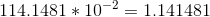Notice that the negative power has no influence on the sign of the number. This allows you to eliminate the negative options. The correct answer is:When you move the decimalplaces to the right, you also get: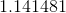2 Next →